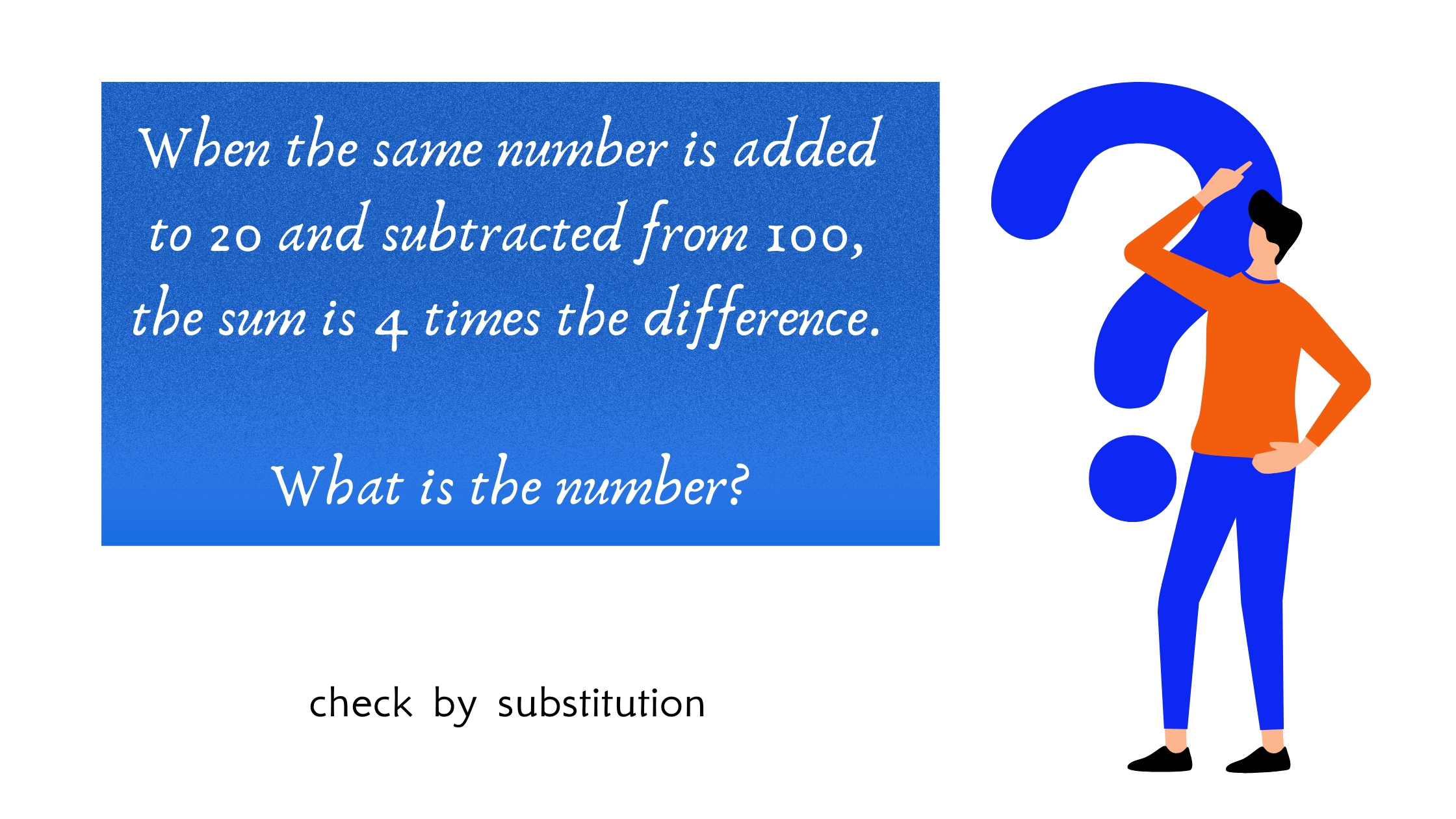# Equations. Linear Equations. Equation PropertiesAn equation is a statement that the two quantities (expressed algebraically) are equal.

An equation always has an equal sign.

To solve an equation means to determine the value of the variable (unknown) that makes two sides of the equation equal.

Equations have existed for a very long time and were first invented by a Persian mathematician Muhammad ibn Musa al-Khwarizmi.

Al-Khwarizmi  defined two basic processes that helped solve equations:

• al-jabr – the process of removing negative terms from an equation
• al-muqabala – the process of reducing positive terms of the same power when they appear on both sides of an equation

Depending on the relationship between the two quantities, equations could be of different types: linear, quadratic, exponential, etc.

A solution of an equation is also called a root.

Decide, which ones of the following are equations:Welcome to your Equation or Not Quiz!

## Linear Equations and Their Properties

Linear equations are equations of the first order (the degree/highest exponent of a variable is 1).

When solving a linear equation one could get three possible outcomes:

• Infinitely many solutions (for example, )
• No solutions (for example, )
• One solution (for example, )

The value of the root of the equation will not change if you move one of the terms of the equation from one side to another while changing the sign of the term to the opposite.

Try solving the following equation (answer: ):

The value of the root of the equation will not change if you multiply or divide both sides of the equation (every term) by the same number except zero.

Solve the following equations:

## An Equation Challenge from the Ancient Greece

Diophantus of Alexandria was a Greek mathematician who lived around the 3rd century CE.

He came up with a solution to the problem below and derived a general equation for it, which was later named after him.

Diophantine equations” mean equations with integer coefficients, the solutions to which are to be found among integers.

Try our Free Online Math Quizzes

1